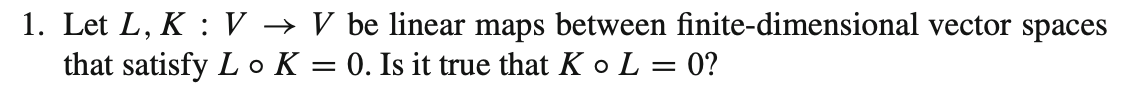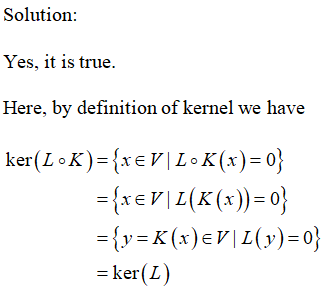# 1. Let L, K : V → V be linear maps between finite-dimensional vector spacesthat satisfy L o K = 0. Is it true that K o L = 0?

Question
1 views

(Proving Linear Algebra)help_outlineImage Transcriptionclose1. Let L, K : V → V be linear maps between finite-dimensional vector spaces that satisfy L o K = 0. Is it true that K o L = 0? fullscreen
check_circle

Solution:...

### Want to see the full answer?

See Solution

#### Want to see this answer and more?

Solutions are written by subject experts who are available 24/7. Questions are typically answered within 1 hour.*

See Solution
*Response times may vary by subject and question.
Tagged in

### Math